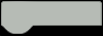I have set up the liquid level sensor with an Arduino, a current to voltage converter  and a 24V DC power supply.

But this scheme returns several different levels:

depth:197.92mm
depth:338.54mm
depth:1075.52mm
depth:338.54mm
depth:796.88mm
depth:184.90mm
depth:171.88mm
depth:171.88mm
depth:364.58mm
depth:1036.46mm
depth:286.46mm....

CODE:

#define ANALOG_PIN A1
#define RANGE 5000 // Depth measuring range 5000mm (for water)
#define CURRENT_INIT 4.00 // Current @ 0mm (uint: mA)
#define DENSITY_WATER 1 // Pure water density normalized to 1
#define DENSITY_GASOLINE 0.74 // Gasoline density
#define PRINT_INTERVAL 1000

int16_t dataVoltage;
float dataCurrent, depth; //unit:mA
unsigned long timepoint_measure;

void setup()
{
Serial.begin(9600);
pinMode(ANALOG_PIN, INPUT);
timepoint_measure = millis();
}

void loop()
{
if (millis() - timepoint_measure > PRINT_INTERVAL) {
timepoint_measure = millis();

dataCurrent = dataVoltage / 120.0; //Sense Resistor:120ohm
depth = (dataCurrent - CURRENT_INIT) * (RANGE/ DENSITY_WATER / 16.0); //Calculate depth from current readings

if (depth < 0) depth = 0.0;

//Serial print results
Serial.print(“depth:”);
Serial.print(depth);
Serial.println(“mm”);
}
}